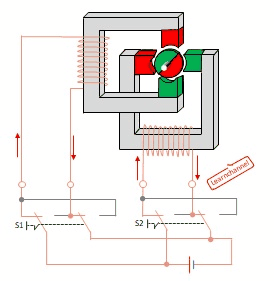## Stepper motor - have a closer looksymbol stepper motor

Stepper motors are often used for positioning without using a sensor, as the actual position can be determined by the specific step angle. Also can this motor apply its full moment even at standstill, without overheating. Or to tell it in other words: its holding torque is the same as its nominal torque. But how does this motor can achieve these outstanding features?

Symbol stepper motor according EN 60617:

How does a stepper motor work?

Here you see a very simple model of a permanent-magnet bipolar stepping motor, which is controlled in full step mode. In the following we will explain these terms. In total, you have to switch 4 times until this motor has completed a full 360 ° turn.Stepper motor - how it works

Parameters which determine the structure of a stepping motor - and thus also its step angle α are:
=> m = Number of stator phases
=> Nr = Number of rotor poles or teeth

The magnitude of step angle for stepper motor is given by:
α =   360°
2 m Nr

Steps per revolution is given i.e. resolution:  = 360°  =    360°
α            2 m Nr

step frequency or Steps per second = rpm * steps per revolution
60 s/min

Example:   A stepper motor with 2 stator phases and a 4-pole rotor has a rotates at 720 rpm. Calculate
a) the step angle
b) the number of steps per revolution
c) Step frequency